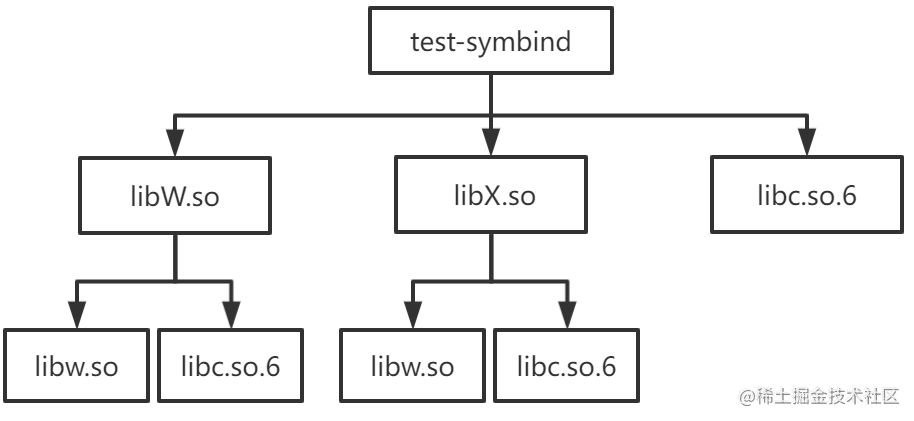# 深入浅出 Sanitizer Interceptor 机制

## Sanitizer 简介

Sanitizer 是由 Google 开源的一系列动态代码分析工具，从 Clang 3.1 和 GCC 4.8 开始被集成在 Clang 和 GCC 中，能够帮助程序员快速准确地在运行时定位程序中的内存错误和多线程错误。Sanitizer 工具集包括：

• LeakSanitizer (LSan)：用于检测内存泄漏
• UndefinedBehaviorSanitizer (UBSsan)：用于检测未定义行为
• MemorySanitizer (MSan)：用于检测未初始化内存的访问

• ASan 编译时会在每一处内存读写语句之前插入代码，根据每一次访问的内存所对应的影子内存 ( shadow memory，就是使用额外的内存来记录常规的内存状态）的状态来检测本次内存访问是否合法。还会在栈变量和全局变量附近申请额外内存作为危险区，用于检测内存溢出。
• ASan 运行时库会替换 malloc/free, operator new/delete 等内存分配函数的实现，这样应用程序的内存分配都由 ASan 实现的内存分配器负责。ASan 内存分配器会在它分配的堆内存附近申请额外内存用于检测堆内存溢出，还会将被释放的内存优先放在隔离区 (quarantine) 用于检测像 heap-use-after-free 这样的堆内存错误。

## Symbol interposition

1. 一个最简单的方式就是在我们的应用程序中定义一个同名的 malloc 函数
2. 还有一种方式就是将我们的 malloc 函数实现在 libmymalloc.so 中，然后在运行我们的应用程序之前设置环境变量 `LD_PRELOAD=/path/to/libmymalloc.so`

When resolving symbolic references, the dynamic linker examines the symbol tables with a breadth-first search. That is, it first looks at the symbol table of the executable program itself, then at the symbol tables of the `DT_NEEDED` entries (in order), and then at the second level `DT_NEEDED` entries, and so on.

``````\$ cat main.c
extern int W(), X();

int main() { return (W() + X()); }

\$ cat W.c
extern int b();

int a() { return (1); }
int W() { return (a() - b()); }

\$ cat w.c
int b() { return (2); }

\$ cat X.c
extern int b();

int a() { return (3); }
int X() { return (a() - b()); }

\$ cat x.c
int b() { return (4); }

\$ gcc -o libw.so -shared w.c
\$ gcc -o libW.so -shared W.c -L. -lw -Wl,-rpath=.
\$ gcc -o libx.so -shared x.c
\$ gcc -o libX.so -shared X.c -L. -lx -Wl,-rpath=.
\$ gcc -o test-symbind main.c -L. -lW -lX -Wl,-rpath=.

````````````\$ LD_DEBUG="symbols:bindings" ./test-symbind
1884890:        symbol=a;  lookup in file=./test-symbind 
1884890:        symbol=a;  lookup in file=./libW.so 
1884890:        binding file ./libW.so  to ./libW.so : normal symbol `a'
1884890:        symbol=b;  lookup in file=./test-symbind 
1884890:        symbol=b;  lookup in file=./libW.so 
1884890:        symbol=b;  lookup in file=./libX.so 
1884890:        symbol=b;  lookup in file=/lib/x86_64-linux-gnu/libc.so.6 
1884890:        symbol=b;  lookup in file=./libw.so 
1884890:        binding file ./libW.so  to ./libw.so : normal symbol `b'
1884890:        symbol=a;  lookup in file=./test-symbind 
1884890:        symbol=a;  lookup in file=./libW.so 
1884890:        binding file ./libX.so  to ./libW.so : normal symbol `a'
1884890:        symbol=b;  lookup in file=./test-symbind 
1884890:        symbol=b;  lookup in file=./libW.so 
1884890:        symbol=b;  lookup in file=./libX.so 
1884890:        symbol=b;  lookup in file=/lib/x86_64-linux-gnu/libc.so.6 
1884890:        symbol=b;  lookup in file=./libw.so 
1884890:        binding file ./libX.so  to ./libw.so : normal symbol `b'

``````
• 函数 a 在 libW.solibX.so 中都有一份定义，但因为是按照 test-symbind, libW.so, libX.so, libc.so.6, libw.so, libx.so 的顺序查找符号定义的，所以最终所有对函数 a 的引用都绑定到 libW.so 中函数 a 的实现
• 函数 b 在 libw.solibx.so 中都有一份定义，但因为是按照 test-symbind, libW.so, libX.so, libc.so.6, libw.so, libx.so 的顺序查找符号定义的，所以最终所有对函数 b 的引用都绑定到 libw.so 中函数 b 的实现

• 方式一：在我们的应用程序中定义一个同名的 malloc 函数。动态链接器在查找符号时 executable 的顺序在 libc.so.6 之前，因此所有对 malloc 的引用都会绑定到 executable 中 malloc 的实现。
• 方式二：将我们的 malloc 函数实现在 libmymalloc.so 中，然后在运行我们的应用程序之前设置环境变量 LD_PRELOAD=/path/to/libmymalloc.so。动态链接器在查找符号时 libmymalloc.so 的顺序在 libc.so.6 之前，因此所有对 malloc 的引用都会绑定到 libmymalloc.so 中 malloc 的实现。

``````// test.cpp
#include <iostream>
int main() {
}

``````

``````\$ objdump -p test-gcc-asan | grep NEEDED
NEEDED               libasan.so.5
NEEDED               libstdc++.so.6
NEEDED               libm.so.6
NEEDED               libgcc_s.so.1
NEEDED               libc.so.6

``````

``````\$ LD_DEBUG="bindings" ./test-gcc-asan
3309213:        binding file /lib/x86_64-linux-gnu/libc.so.6  to /usr/lib/x86_64-linux-gnu/libasan.so.5 : normal symbol `malloc' [GLIBC_2.2.5]
3309213:        binding file /lib64/ld-linux-x86-64.so.2  to /usr/lib/x86_64-linux-gnu/libasan.so.5 : normal symbol `malloc' [GLIBC_2.2.5]
3309213:        binding file /usr/lib/x86_64-linux-gnu/libstdc++.so.6  to /usr/lib/x86_64-linux-gnu/libasan.so.5 : normal symbol `malloc' [GLIBC_2.2.5]

``````

## Sanitizer interceptor

Sanitizer interceptor 存在一个测试文件interception_linux_test.cpp

``````#include "interception/interception.h"
#include "gtest/gtest.h"

static int InterceptorFunctionCalled;

DECLARE_REAL(int, isdigit, int);

INTERCEPTOR(int, isdigit, int d) {
++InterceptorFunctionCalled;
return d >= '0' && d <= '9';
}

namespace __interception {

TEST(Interception, Basic) {
EXPECT_TRUE(INTERCEPT_FUNCTION(isdigit));

// After interception, the counter should be incremented.
InterceptorFunctionCalled = 0;
EXPECT_NE(0, isdigit('1'));
EXPECT_EQ(1, InterceptorFunctionCalled);
EXPECT_EQ(0, isdigit('a'));
EXPECT_EQ(2, InterceptorFunctionCalled);

// Calling the REAL function should not affect the counter.
InterceptorFunctionCalled = 0;
EXPECT_NE(0, REAL(isdigit)('1'));
EXPECT_EQ(0, REAL(isdigit)('a'));
EXPECT_EQ(0, InterceptorFunctionCalled);
}

}  // namespace __interception

``````

• `INTERCEPTOR(int, isdigit, int d) { ... }` 用于将函数 `isdigit` 的实现替换为 { ... } 的实现
• 在代码中调用 `isdigit` 之前，需要先调用 `INTERCEPT_FUNCTION(isdigit)`。如果 `INTERCEPT_FUNCTION(isdigit)` 返回为 true，则说明成功替换了将 libc 中 `isdigit` 函数的实现。
• `REAL(isdigit)('1')` 用于调用真正的 `isdigit` 实现，不过在调用 `REAL(isdigit)('1')` 之前需要先 `DECLARE_REAL(int, isdigit, int)`

``````INTERCEPTOR(int, isdigit, int d) {
++InterceptorFunctionCalled;
return d >= '0' && d <= '9';
}

INTERCEPT_FUNCTION(isdigit);

DECLARE_REAL(int, isdigit, int);
REAL(isdigit)('1');

``````
• 我们首先看下 INTERCEPTOR 宏做了哪些事情

• 首先在 __interception namespace 中定义了一个函数指针 real_isdigit，该函数指针实际上在 INTERCEPT_FUNCTION 宏中会被设置为指向真正的 `isdigit` 函数地址。
• 然后将 `isdigit` 函数设置为弱符号 (weak)，并且将 `isdigit` 设置成 `__interceptor_isdigit` 的别名 (alias)。
• 最后将我们自己版本的 `isdigit` 函数逻辑实现在 `__interceptor_isdigit` 函数中

``````extern "C" int __interceptor_isdigit(int d);
extern "C" int isdigit(int d) {
fprintf(stderr, "my_isdigit_interceptor\n");
return __interceptor_isdigit(d);
}

``````

• 接着我们看下 INTERCEPT_FUNCTION 宏做了哪些事情

• INTERCEPT_FUNCTION 宏展开后就是对 __interception::InterceptFunction 函数的调用。`InterceptFunction`函数定义
``````namespace __interception {
// If the lookup using RTLD_NEXT failed, the sanitizer 运行时库 is
// later in the library search order than the DSO that we are trying to
// intercept, which means that we cannot intercept this function. We still
// want the address of the real definition, though, so look it up using
// RTLD_DEFAULT.

// In case `name' is not loaded, dlsym ends up finding the actual wrapper.
// We don't want to intercept the wrapper and have it point to itself.
}
}

bool InterceptFunction(const char *name, uptr *ptr_to_real, uptr func,
uptr wrapper) {
return addr && (func == wrapper);
}
}  // namespace __interception

``````

• dlsym 的第一个参数为 RTLD_DEFAULT 时，查找名为 name 的函数地址的顺序就是前面提到的 executable, preload0.so, preload1.so needed0.so, needed1.so, needed2.so, needed0_of_needed0.so, needed1_of_needed0.so, ... 这个顺序。

• dlsym 的第一个参数为 RTLD_NEXT 时，则是以当前 object 后面动态库为起点去查找名为 name 的函数的地址

• 最后我们看下 DECLARE_REAL 宏 和 REAL 宏做了哪些事情

DECLARE_REAL 展开后就是声明了在 __interception namespace 中存在一个指向被替换函数真正实现的函数指针，REAL 宏就是通过这个函数指针来调用被替换函数的真正实现。

## 总结

ASan 基于 sanitizer interceptor 机制替换了 malloc/free 这类的内存分配/释放函数，使得所有的内存分配和释放都由 ASan 实现的内存分配器负责，这样 ASan 就能很容易检测到 heap-use-after-free，double-free 这样的堆内存错误。

## 直播预告